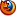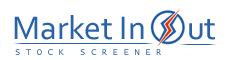Please enable JavaScript to view this page content properly Log In | Sign Up
 Pre-Tax Profit Margin Leverage Ratio Current Ratio Price / Book Ratio Price / Tangible Book Ratio Price / Cash Flow Ratio Price / Free Cash Flow Ratio Debt / Equity Ratio Price / Sales Ratio Income per Employee
 Price / Book Ratio Action Price / Book Ratio: More than 20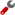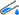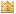Price / Book Ratio: 10 to 20Price / Book Ratio: 8 to 10Price / Book Ratio: 5 to 8Price / Book Ratio: 3 to 5Price / Book Ratio: 2 to 3Price / Book Ratio: 1 to 2Price / Book Ratio: 0 to 1Best viewed in